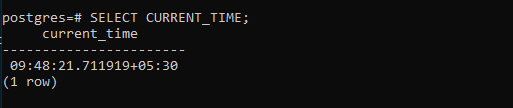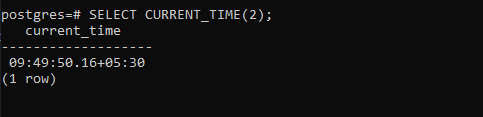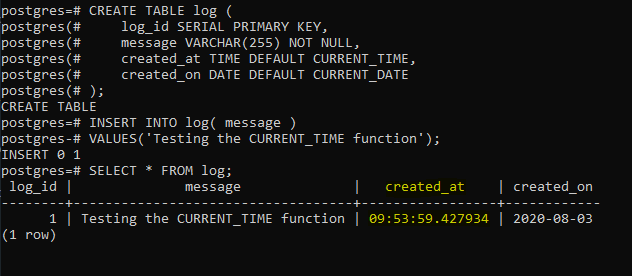Related Articles

# PostgreSQL – CURRENT_TIME Function

• Last Updated : 01 Feb, 2021

The PostgreSQL CURRENT_TIME function returns the current time and the currentthe time zone.

```Syntax: CURRENT_TIME(precision)
```

Let’s analyze the above syntax:

• The precision argument is used to set the precision of the returned TIMESTAP type value in fractional seconds precision. By default the function returns a full available precision if not precision data is provided to the function.
• The CURRENT_TIME function returns a TIME WITH TIME ZONE value. This value is nothing but the current time with the the current time zone.

Example 1:

The following statement can be used to get the current time:

```SELECT CURRENT_TIME;
```

Output:Example 2:

The following statement shows the process of using the CURRENT_TIME function with the precision of 2:

```SELECT CURRENT_TIME(2);
```

Output:Example 3:

The CURRENT_TIME function can also be used as the default value of the TIME columns. To demonstrate this, create a table named log:

```CREATE TABLE log (
log_id SERIAL PRIMARY KEY,
message VARCHAR(255) NOT NULL,
created_at TIME DEFAULT CURRENT_TIME,
created_on DATE DEFAULT CURRENT_DATE
);
```

The log table has the created_at column whose default value is the value returened by the CURRENT_TIME function. Now insert some data to the demo table:

```INSERT INTO log( message )
VALUES('Testing the CURRENT_TIME function');
```

Now verify if the row was inserted into the log table with the created_at column added correctly by using the following query:

```SELECT * FROM log;
```

Output:My Personal Notes arrow_drop_up